# Negative Numbers on a Number Line

Negative Numbers on a Number Line• The numbers to the right of zero on a number line are called positive numbers.
• Positive numbers are greater than zero and get larger as we move further to the right on the number line.
• Negative numbers are to the left of zero on a number line.
• We mark negative numbers with a negative sign ‘-‘ to show that they are negative.
• The of the number itself tells us how many places away from zero that this number is.
• If the numeral has a negative sign in front of it, it is this number of places left of zero.
• We don’t tend to write a positive sign ‘+’ in front of a positive number.
• Therefore, if a number has no sign in front of it, it is a positive number.
• Zero is neither positive nor negative.
Positive numbers are to the right of zero and negative numbers are to the left of zero, marked with a negative sign: ‘-‘.

The numeral tells us how far away from zero the number is.• Here we have the example of -2 + 8.
• When we add, we move to the right on a number line.
• When we subtract we move to the left on a number line.
• We start at -2, which is two places to the left of zero.
• The plus sign means that we move to the right.
• We are adding 8, which means we move to the right 8 places.
• We arrive at 6.
• Therefore -2 + 8 = 6.Supporting Lessons# Negative Numbers on a Number Line

## What are Negative Numbers?

Negative numbers are numbers that are less than zero. They are left of zero on a number line. Negative numbers are used to show when something is going in the other direction to other numbers. We write a minus sign ‘-‘ in front of a number to show that it is negative.

Negative numbers are used in real life to show temperature, money owed and levels of a building below the ground floor.

On a number line, positive numbers are numbers that are to the right of zero. A positive number is any number that is larger than zero.

We can write a positive sign ‘+’ in front of a number to show that it is positive, however we do not do this unless we need to.

If a number does not have a sign in front of it, then it is a positive number.The positive numbers get larger as we move to the right.

Negative numbers are to the left of zero.Going from right to left, we can also count upwards from zero. However, to show that the numbers are negative, we write a minus sign: ‘-‘ in front of each number.Regardless of whether a number is positive or negative, the size of each

tells us how far away the number is from zero.

The numeral of a positive number tells us how many places right of zero it is. If there is a negative sign ‘-‘ in front then the numeral tells us how many places to the left of zero it is.

Negative numbers are commonly used in the following real-life situations:

• Temperature: Negative numbers are used to show colder temperatures below 0°. The larger the negative number numeral, the colder the temperature.
• Money: Negative numbers are used to show money owed or money transferred out. For example -$20 can mean that$20 is owed. It could also show that \$20 has been spent.
• Floors of a Building: Negative numbers are used to show floors below the ground floor. The larger the negative number numeral, the further below ground the floor is.

## Addition and Subtraction on a Number Line with Negatives

To add a number, we move this many places right on the number line. To subtract a number, we move this many places left on the number line.

Here is an example of subtracting a larger number than we started with.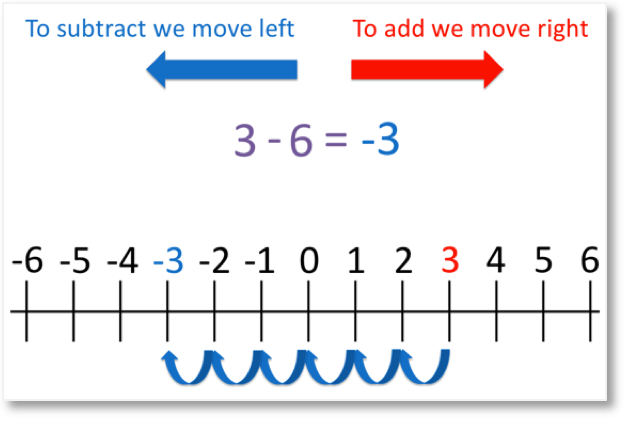To calculate 3 – 6, first find 3 on the number line. This has been highlighted in red.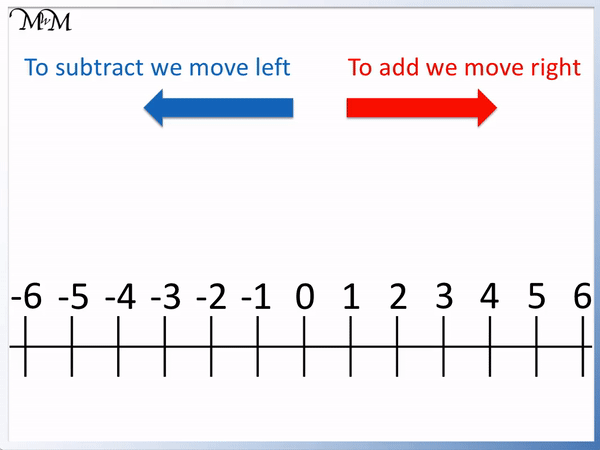We are subtracting 6, so we move 6 places to the left from 3.

We arrive at – 3   (negative three).

Therefore,

3 – 6 = – 3.

In this example we subtracted a larger number from a smaller number. When we lose more than we start with, we end up with a negative number.

We could have worked out 3 – 6 since we know that 6 – 3 = 3. If 6 – 3 = 3, then 3 – 6 = -3. Our answer is the same numeral but instead it is negative.

When adding a negative number to a positive number, the size of the numbers determines whether the answer is positive or negative. If the positive number is larger than the negative number, then the answer is positive. If the negative number is larger than than the positive number, the answer is negative.

Here is another example of subtracting a larger number from a smaller number. We have 1 – 3. We know that the answer will be negative because we are subtracting a number that is larger than the number we start with.To calculate 1 – 3, we start at 1 on the number line.

We want to subtract 3, so we move 3 places to the left.1 – 3 = – 2.

Again since we know 3 – 1 = 2, we know that 1 – 3 = -2. The same numeral but a negative answer since we are subtracting a number that is larger than we started with.

Here is an example of a sum that starts with a negative number. We have a negative number add a positive number. We have -2 + 8.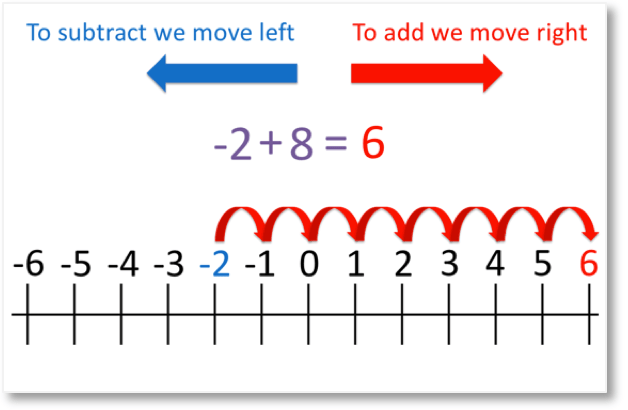We begin by finding –2 on the number line.

We want to add 8, so we move 8 places to the right.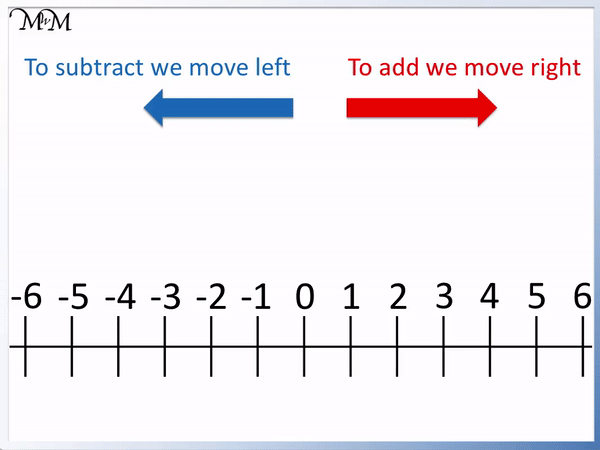We arrive at 6.

Therefore,

–2 + 8 = 6.

Since the positive number was larger than the negative number, the answer was positive.

We can work out additions with negative numbers more easily by rearranging the sum so that the positive number comes first.

For example -2 + 8 can be rearranged to say +8 -2. We know that 8 – 2 = 6 and so, -2 + 8 = 6.

Here is an example of -6 + 4. Here the negative number numeral is larger than the postive number numeral. So we expect to arrive at a negative answer.To calculate –6 + 4, we begin by finding – 6 on the number line.

We want to add 4, so we move 4 places to the right.

We arrive at –2. We knew that this answer would be negative since we subtracted a larger number than the number we started with.

Therefore,

–6 + 4 = –2.

Since we know that 6 – 4 = 2, we know that 4 – 6 = -2. When we switch the numbers in the subtraction, the sign of the answer changes from positive to negative.

Here’s our final example with two negative signs in the sum:We are asked to calculate – 1 – 4. We begin by finding – 1 on the number line.

We want to subtract 4, so we move 4 places to the left.We arrive at – 5.

Therefore,

– 1 – 4 = – 5.

We started one place to the left of zero and then moved a further four places left to get to five places left of zero.

## Finding the Difference Between Positive and Negative Numbers

To find the difference between numbers, we subtract the smaller number from the larger number.

To find the difference between a positive and negative number, add the two numerals together as if they were both positive.

To find the difference between two negative numbers, ignore the negative signs and subtract the smallest number from the largest.

Here is an example of finding the difference between two positive numbers, 5 and 2.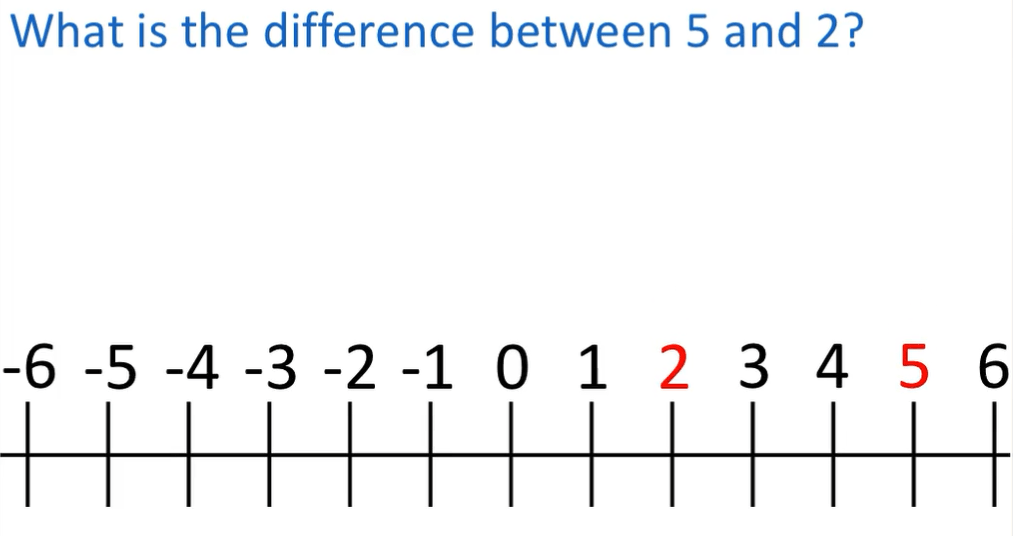We can see this difference on a number line by finding the two numbers and counting the number of jumps between them.

There are 3 jumps between 2 and 5. The difference between 5 and 2 is 3.We can work this out more quickly by subtracting 2 from 5. 5 – 2 = 3.

Here is the same example but both numbers are negative this time.

We wish to find the difference between -5 and -2.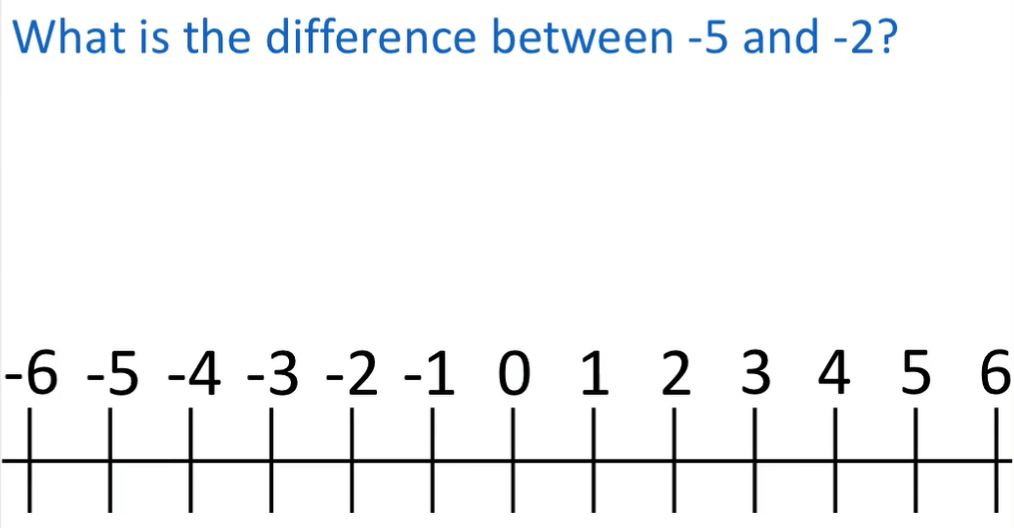We can find -5 and -2 on our number line and then count the number of jumps between the two numbers.

We can see that there are 3 jumps between -5 and -2.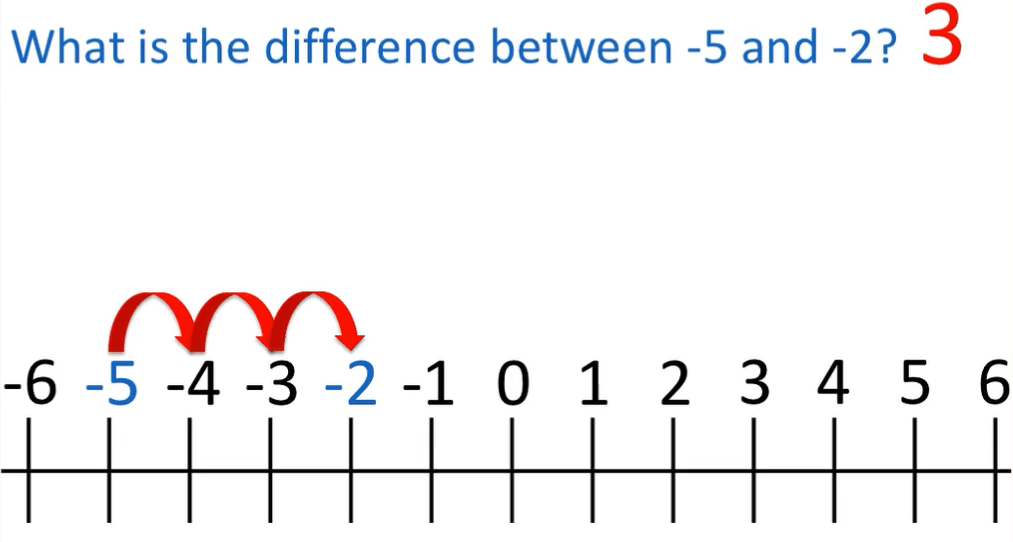We can see that finding the difference between -2 and -5 is very similar to finding the difference between 2 and 5. The numbers are the same distance apart, they are just on the other side of zero.

So to work out the difference between -2 and -5, we can ignore the negative signs and just subtract the smaller number from the larger number.

5 – 2 = 3 and so, the difference between -5 and -2 is 3.

In this example, we find the difference between a negative and a positive number.

We have -3 and 4.We can see that the difference between the numbers is 7. There are 7 jumps between -3 and 4.We can more easily work this out by counting the number of jumps from -3 to 0 and then from 0 to 4.

The numeral of any number tells us how far it is away from zero. So -3 is 3 places to the left of zero and 4 is 4 places to the right of 0.

We can simply ignore the negative signs in the numbers and then just add them together to find the difference between them.

3 + 4 = 7 and so the difference between -3 and 4 is 7.Now try our lesson on Subtracting a Negative Number from a Negative Number where we learn how to subtract a negative number.error: Content is protected !!